Graphing Tidbits
Both Classic Mode and MathPrint Mode

 1.  To find the y-intercept:         Hit the TRACE button.  The spider will always start on the y-axis, showing the y-intercept.2.  To find the x-intercept:          Hit 2nd TRACE button, #2 ZERO (called the "Root Key") allows you to find the x-intercept(s).        When asked for the Left Bound, move the cursor to the left of the intercept.  Hitting ENTER will place a marker on the screen.  For the Right Bound, move to the right of the intercept.  Hit ENTER twice.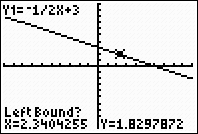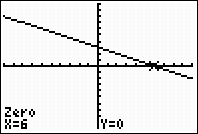3.  To find where the graphs intersect:         Hit 2nd TRACE button, #5 INTERSECT.       When asked for the First Curve, move the cursor near the point of intersection you wish to find.  Simply hit ENTER three times.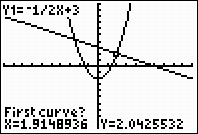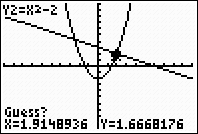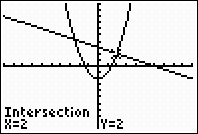4.  To find a specific value while tracing:       When tracing a graph, you may not be able to find the specific value that you desire by  moving the arrow keys.  To find your specific value while tracing, simply type the x-value that you are looking for and hit ENTER.  The cursor will automatically move to that point and yield the y-value, if the x-value is within the viewing window.  If your needed x-value is outside of the viewing window, adjust the window as to contain the needed x-value.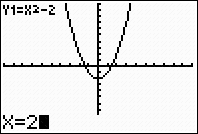5.  To enter multiple graphs quickly:      Lists can be used to enter several equations with similar coefficients or constants.  Be sure to use French curly braces and not the parentheses.  For example, Y1 = x + {3, 4, 5} will plot y = x + 3, y = x + 4, and y = x + 5.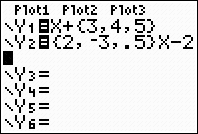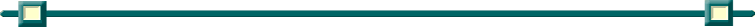Finding Your Way Around TABLE of  CONTENTS